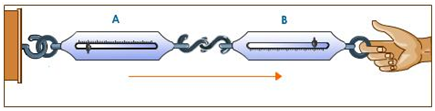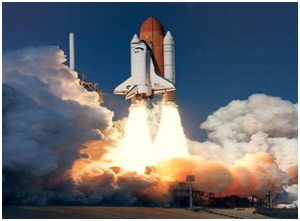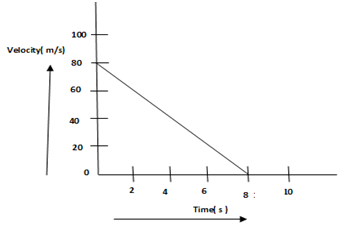# MCQ Questions for Class 9 Science Chapter 9 Force and Laws of Motion with Answers

## MCQ Questions for Class 9 Science Chapter 9 Force and Laws of Motion with Answers

MCQs from Class 9 Science Chapter 9 – Force and Laws of Motion are provided here to help students prepare for their upcoming Science exam.

MCQs from CBSE Class 9 Science Chapter 9: Force and Laws of Motion

1. A goalkeeper in a game of football pulls his hands backwards after holding the ball shot at the goal. This enables the goalkeeper to:
(a) Exert large force on the ball
(b) Increases the force exerted by the ball on hands
(c) Increase the rate of change of momentum
(d) Decrease the rate of change of momentum

(d) Decrease the rate of change of momentum

2. An object of mass 2 kg is sliding with a constant velocity of 4 m/s on a friction less horizontal table. The force required to keep the object moving with the same velocity is:
(a) 32 N
(b) 0 N
(c) 2 N
(d) 8 N

(b) 0 N

3. Newton’s third law of motion explains the two forces namely ‘action’ and ‘reaction’ coming into action when the two bodies are in contact with each other. These two forces:(a) Always act on the same body
(b) Always act on the different bodies in opposite directions
(c) Have same magnitude and direction
(d) Acts on either body at normal to each other

(b) Always act on the different bodies in opposite directions

4. In a rocket, a large volume of gases produced by the combustion of fuel is allowed to escape through its tail nozzle in the downward direction with the tremendous speed and makes the rocket to move upward.Which principle is followed in this take off of the rocket?
(a) Moment of inertia
(b) Conservation of momentum
(c) Newton’s third law of motion
(d) Newton’s law of gravitation

(b) Conservation of momentum

5. A water tank filled upto 2/3 of its height is moving with a uniform speed. On sudden application of the brake, the water in the tank would
(a) Move backward
(b) Move forward
(c) Come to the rest
(d) Be unaffected

(b) Move forward

6. Velocity versus time graph of a ball of mass 50 g rolling on a concrete floor is shown in the figure below. What will be the frictional force of the floor on the ball?(a) 0.5 N
(b) 50 N
(c) 5 N
(d) 0.05 N

(a) 0.5 N

7. The seat belts are provided in the cars so that if the car stops suddenly due to an emergency braking, the persons sitting on the front seats are not thrown forward violently and saved from getting injured. Can you guess the law due to which a person falls in forward direction on the sudden stopping of the car?
(a) Newton’s first law of motion
(b) Newton’s second law of motion
(c) Newton’s third law of motion
(d) Newton’s law of gravitation

(a) Newton’s first law of motion

8. When a balloon held between the hands is pressed, its shape changes. This happens because:
(a) Balanced forces act on the balloon
(b) Unbalanced forces act on the balloon
(c) Frictional forces act on the balloon
(d) Gravitational force acts on the balloon

(a) Balanced forces act on the balloon

9. Which of the following situations involves the Newton’s second law of motion?
(a) A force can stop a lighter vehicle as well as a heavier vehicle which are moving
(b) A force exerted by a lighter vehicle on collision with a heavier vehicle results in both the (vehicles coming to a standstill
(c) A force can accelerate a lighter vehicle more easily than a heavier vehicle which are moving
(d) A force exerted by the escaping air from a balloon in the downward direction makes the balloon to go upwards

(c) A force can accelerate a lighter vehicle more easily than a heavier vehicle which are moving

10. The speed of a car weighing 1500 kg increases from 36 km/h to 72 km/h uniformly. What will be the change in momentum of the car?
(a) 15000 kg km/h
(b) 15000 kg m/s
(c) 54000 kg m/s
(d) 54000 g m/s

(b) 15000 kg m/s

11. A passenger in a moving train tosses a coin which falls behind him. Observing this statement what can you say about the motion of the train?
(a) Accelerated
(b) Retarded
(c) Along circular tracks
(d) Uniform

(a) Accelerated

12. Newton’s first law of motion says that a moving body should continue to move forever , unless some external forces act on it. But a moving cycle comes to rest after some time if we stop pedaling it. Can you choose the correct reason for the stoppage of cycle?
i. Air resistance
ii. Gravitational pull of the earth
iv. Heat of the environment
Choose the correct option:
(a) (iii) and (iv)
(b) (i) and (iii)
(c) (i) and (ii)
(d) (ii) and (iii)

(b) (i) and (iii)

13. A man wearing a bullet-proof vest stands on roller skates. The total mass is 80 kg. A bullet of mass 20 g is fired at 400 m/s. It is stopped by the vest and falls to the ground. What is then the velocity of the man?
(a) 1 m/s
(b) 0.1 m/s
(c) 0.01 m/s
(d) 0 m/s

(b) 0.1 m/s

14. The unit of measuring the momentum of a moving body is:
(a) m/s
(b) kg.m/s
(c) kg.m/s2
(d) N m2/kg2

(b) kg.m/s

15. The inertia of a moving object depends on:
i. Mass of the object
ii. Momentum of the object
iii. Speed of the object
iv. Shape of the object

Choose the correct option:
(a) (i) and (ii)
(b) only (i)
(c) only (ii)
(d) (iii) and (iv)